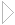Lecture 3 - Page 42 : 42
 Functional Programming in SchemeName binding, Recursion, Iteration, and Continuations * Name binding constructs The let name binding expression The equivalent meaning of let Examples with let name binding The let* name binding construct An example with let* The letrec namebinding construct LAML time functions * Conditional expressions Conditional expressions Examples with if Example with cond: leap-year? Example with cond: american-time Example with cond: as-string * Recursion and iteration Recursion List processing Tree processing (1) Tree processing (2) Recursion versus iteration Example of recursion: number-interval Examples of recursion: string-merge Examples with recursion: string-of-char-list? Exercises * Example of recursion: Hilbert Curves Hilbert Curves Building Hilbert Curves of order 1 Building Hilbert Curves of order 2 Building Hilbert Curves of order 3 Building Hilbert Curves of order 4 A program making Hilbert Curves * Continuations Introduction and motivation The catch and throw idea A catch and throw example The intuition behind continuations Being more precise The capturing of continuations Capturing, storing, and applying continuations Use of continuations for escaping purposes Practical example: Length of an improper list Practical example: Searching a binary tree
 Practical example: Searching a binary tree
 Searching a binary tree involves a recursively defined tree traversalIf we find the node we are looking for it is convenient to throw the out of the tree traversal
 ```(define (find-in-tree tree pred) (call-with-current-continuation (lambda (found) (letrec ((find-in-tree1 (lambda (tree pred) (if (pred tree) (found tree) (let ((subtrees (subtree-list tree))) (for-each (lambda (subtree) (find-in-tree1 subtree pred)) subtrees))) #f))) (find-in-tree1 tree pred))) ))```

A tree search function which uses a continuation found if we find what we search for. Notice that this examples requires the function subtree-list, in order to work. The function returns #f in case we do not find node we are looking for. Notice that it makes sense in this example to have both the if expression and the #f value in sequence!